Question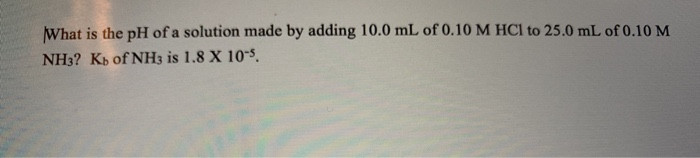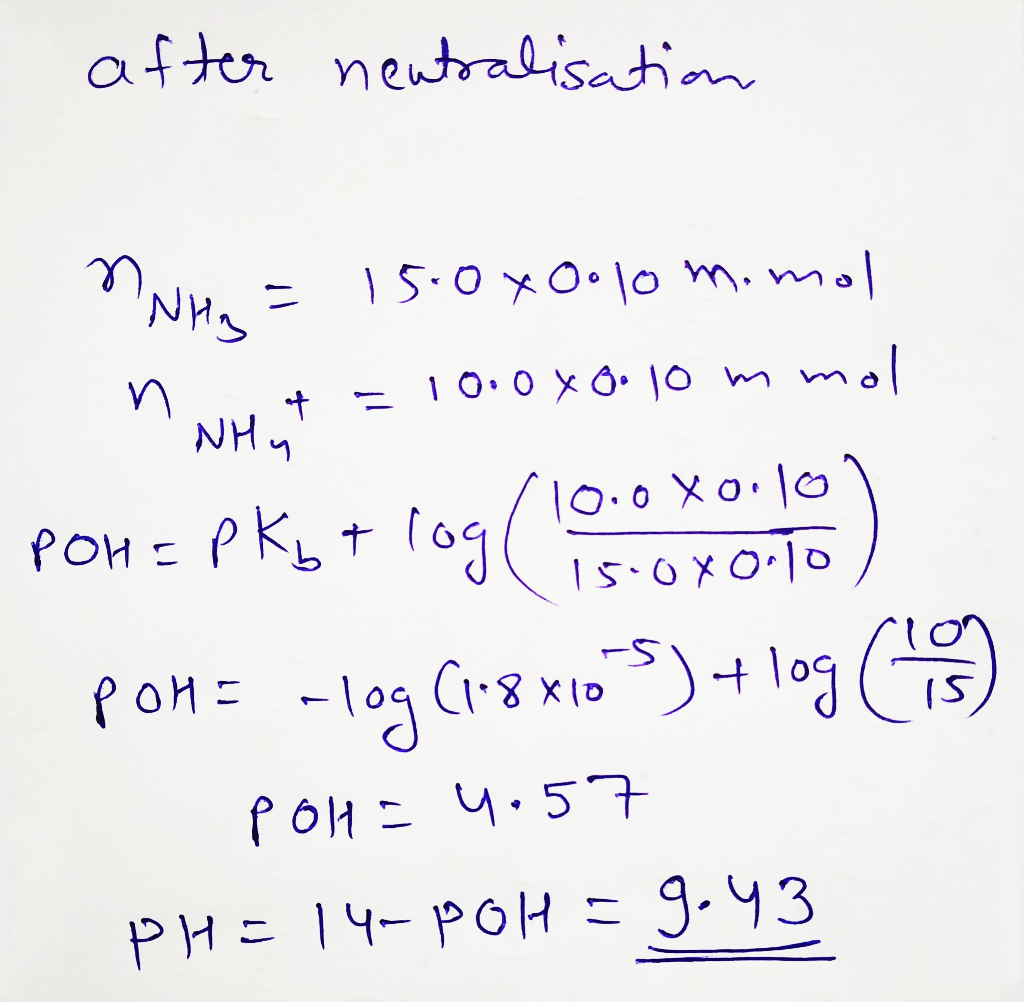#### Earn Coins

Coins can be redeemed for fabulous gifts.

Similar Homework Help Questions
• ### What is the pH of the solution from adding 25 mL of 0.33 M HCl to...

What is the pH of the solution from adding 25 mL of 0.33 M HCl to 25 mL of 0.58 M NH3? (Kb for NH3 is 1.8 x 10^-5)

• ### 4. Calculate the pH of a buffer solution made by adding 500 mL of 0.40 M...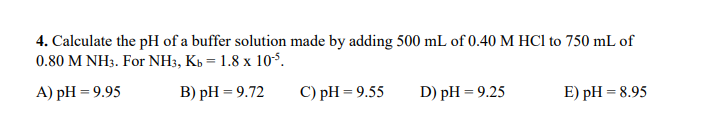4. Calculate the pH of a buffer solution made by adding 500 mL of 0.40 M HCl to 750 mL of 0.80 M NHs. For NH3, Kb-1.8 x 10-5. A) pH-9.95 B) pH -9.72 C)pH -9.55D) pH 9.25 E) pH-8.99

• ### Calculate the pH of a solution prepared by adding 20.0 mL of 0.100 M HCl to...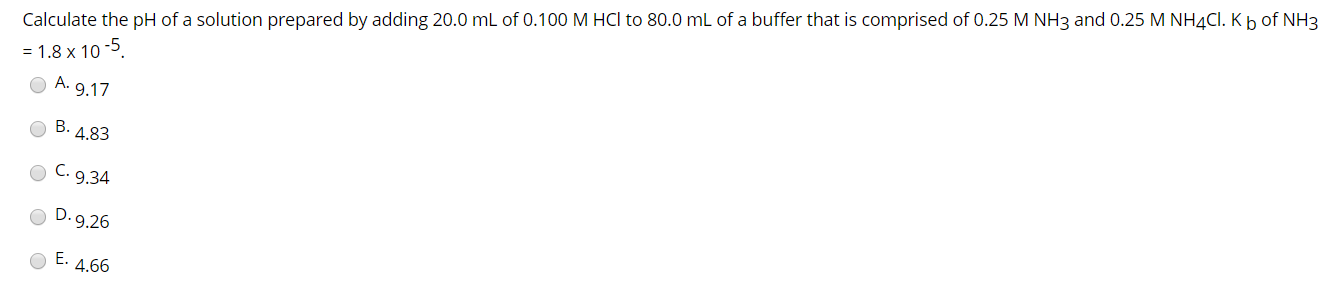Calculate the pH of a solution prepared by adding 20.0 mL of 0.100 M HCl to 80.0 mL of a buffer that is comprised of 0.25 M NH3 and 0.25 M NH4Cl. Kb of NH3 = 1.8 x 10-5. ОА. 9.17 ОВ. 4.83 0 o С. 9.34 OD.9.26 OE. 4.66

• ### A solution prepared by mixing 100 mL of 0.10 M NaOH 140 mL of 0.10 M...

A solution prepared by mixing 100 mL of 0.10 M NaOH 140 mL of 0.10 M HCl 100 mL of 0.10 M NH3 has a pH of: Kb (NH3) = 1.8 x 10-5 A. 9.08 B. 4.57 C. 7.00 D. 4.92 E. 9.43

• ### A 10.0 mL sample of 0.25 M NH3(aq) is titrated with 0.20 M HCl(aq) (adding HCl to NH3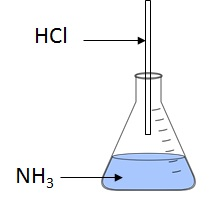1)A 10.0 mL sample of 0.25 M NH3(aq) is titrated with 0.20 M HCl(aq) (adding HCl to NH3). Determine which region on the titration curve the mixture produced is in, and the pH of the mixture at each volume of added acid.Kb of NH3 is 1.8 × 10−5.Henderson–Hasselbalch equation:Part a):1) After adding 10 mL of the HCl solution, the mixture is   [ Select ] ["at", "before", "after"] the equivalence point on the titration curve.2) The pH of the solution after...

• ### (1). The pH of a solution made by mixing 100.0 mL of 0.433 M NH3 with...

(1). The pH of a solution made by mixing 100.0 mL of 0.433 M NH3 with 100.0 mL of 0.259 M HCl is ___.  Kb = 1.8 x 10-5 for NH3.

• ### Question 6 1 pts A 10.0 mL sample of 0.25 M NH3(aq) is titrated with 0.20 M HCl(aq) (adding HCl to NH3). Determine whic...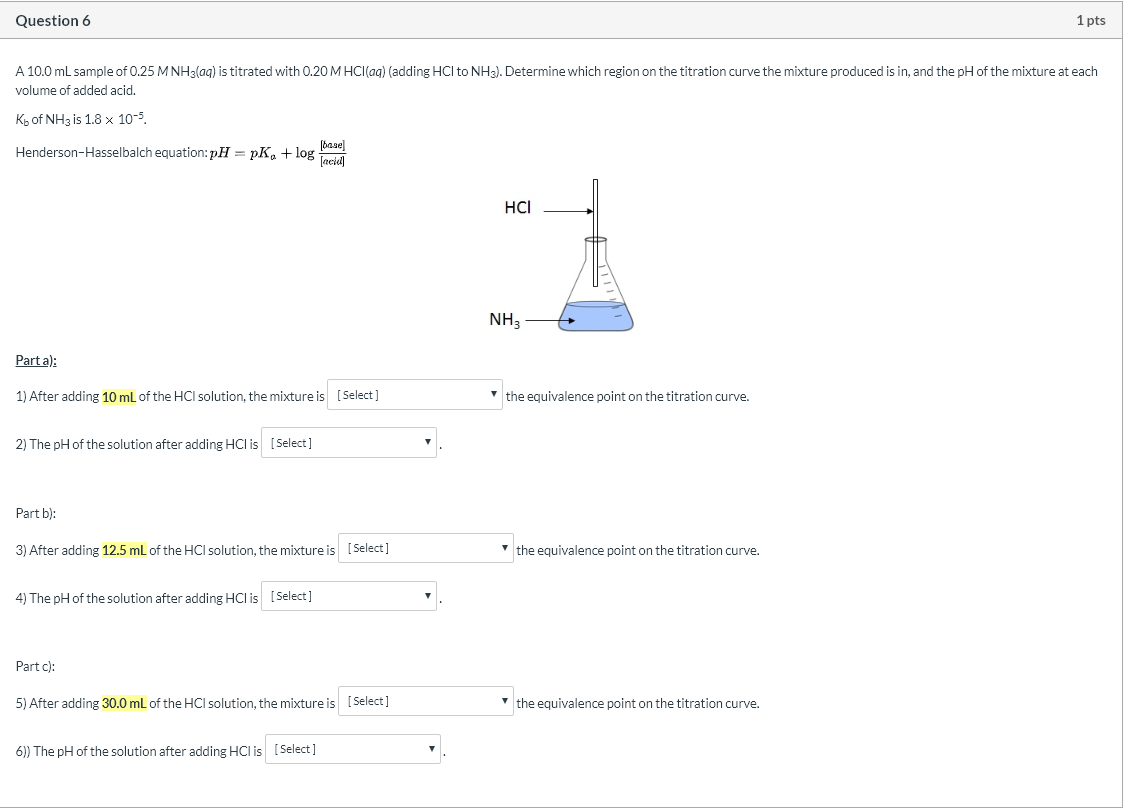Question 6 1 pts A 10.0 mL sample of 0.25 M NH3(aq) is titrated with 0.20 M HCl(aq) (adding HCl to NH3). Determine which region on the titration curve the mixture produced is in, and the pH of the mixture at each volume of added acid. Ko of NH3 is 1.8 x 10-5 Henderson-Hasselbalch equation: pH = pka + log og HCI NH, NH3- Parta): 1) After adding 10 mL of the HCl solution, the mixture is (Select] the equivalence...

• ### What is the pH of a solution prepared by mixing 50.00 mL of 0.10 M NH3...

What is the pH of a solution prepared by mixing 50.00 mL of 0.10 M NH3 with 5.00 mL of 0.10 M NH4Cl? Assume that the volume of the solutions are additive and that Kb = 1.8 × 10-5 for NH3. (Select One) 10.26 or 11.13

• ### Calculate the pH of a solution made by mixing 100.0 mL of 0.874 M NH3 with...

Calculate the pH of a solution made by mixing 100.0 mL of 0.874 M NH3 with 100.0 mL of 0.100 M HCl. (Kb for NH3 = 1.8 10

• ### 1) 7. 10.0 mL of 0.10 M HBr is mixed with 10.0 mL of 0.10 M...

1) 7. 10.0 mL of 0.10 M HBr is mixed with 10.0 mL of 0.10 M HCOOH. What is the pH? 2) 15. Calculate the pH of a solution containing 2.5 x 10 –2 mol of nicotinic acid (a monoprotic acid dissolved in 350 mL of water. ( K a = 1.1 x 10 -5 ) 3) A 1.0 L buffer solution is made up of 0.15M NaF and 0.20 M HF ( pK a = 3.17) . 0.05 mol...